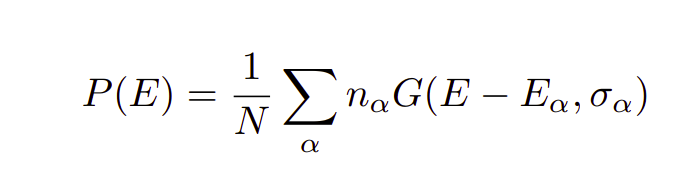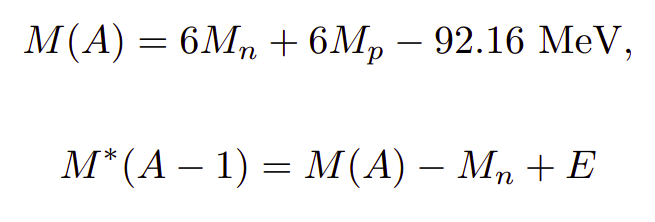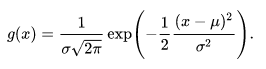# Adding Gaussian PDF in event loop

ROOT Version: 6.14.00
Platform: Ubuntu 20.04 LTS
Compiler: gcc

Dear experts,

I would like to kindly ask for your help.

As a distribution, I want to add a gaussian pdf generated from the given mean(Ea) and sigma. N = sum of na.

From this table,

I want to calculate the total Probability Distribution given using :And find the E distribution from above and add it here:where, M(A) , Mn, Mp are constant values.

Here is my attempted code:

``````#include <string>
#include <iostream>
#include <fstream>
#include <cmath>
#include <vector>
//#include "TH1D.h"
using namespace std;

//Calculate Probability Distribution Function
double norm_pdf(double x, double mu, double sigma, double na)
{

return  na* exp( -((x - mu)*(x-mu))/(2.0*sigma*sigma) );
}

void generateNormPdf(double mu[], double sigma[], double na[], vector<double>& x, vector<double>& density)
{

double N  = 22.0;            // Total number of neutrons (na)
int    nev  =  x.size();       // Number of points to generate between min_range and max_range

double min_range = 0.0;
for (int i = 0; i<7; i++){     // 7 is size of sample
for (int j = 0; j<nev; j++)
{
x[j] = (mu[i]*2.0 - min_range)/(nev-1) * j;
density[j] = (1.0/N)*norm_pdf(x[j], mu[i], sigma[i], na[i]);
}
}

}

int main()
{
int nev = 100;          // Number of samples/events
// PDF
vector<double> x(nev);
vector<double> density(nev);

double mu[]        =  {62.0,40.0,35.0,18.0,13.15,11.45,5.56};    // Mean
double sigma[]     = {6.25,3.75,3.75,1.25,1.0,0.75,0.75};     // Standard Deviation
double na[]        = {2.0,4.0,2.0,2.6,2.0,4.0,2.0};      // # of nucleons
generateNormPdf(mu, sigma, na, x, density);
}
``````

The final value I want is M*(A-1) as distribution (i.e not a constant value).

It would be of great help.

Aren’t you missing the factor `1/sqrt(2pi)/sigma` for the gaussian to be normalized?

Hi! Thanks for the response.
I think the normalization here is N, where N is the total number of “na”.

If you want the normalization to be N, then you need to multiply Na with a normalized pdf. Right now, you are multiplying Na with a function (exp(…)) that is not normalized.

You should use:multiplied by Na.

Okay, Thanks!

Apart from that,

** I want to get the total PDF, my code is incomplete. Any way to extend to get the total probability?

** Is the normalized PDF [i.e P(x) vs x ] the same as a 1D histogram of the “x” weighted by the density?
As I want to add my E (“x” in the code) distribution to M*(A-1) so that I will get a M*(A-1) distribution (not a constant value).

Note: “x means E”.

To get total probabilities:

First, initialize all `density[j]` to zero.

Later, in your loop over ‘i’, change
`density[j] = ...`
with
`density[j] += ...`